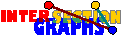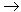# Order

The most important feature of intervals of the real line is their ordering. Of two nonintersecting intervals, one is `to the right' of the other. This relation is obviously irreflexive, asymmetric, and transitive, i.e. a partial order. This carries over to a partial order < of V, distinct vertices x and y of V are adjacent in the intersection graph if and only if they are not comparable by <. That means, G is the cocomparability graph (complement of the comparability graph) of (V,<).

The converse is not true: Not every cocomparability graph of some poset is an interval graph. The reason is that not every poset can be represented by intervals on the real line. Those posets that can are called interval orders.Interval graphs are exactly the cocomparability graphs of interval orders.

But every poset can be represented by graphs of continuous functions f:[0,1]\R, where we have a natural relation `above' for functions with nonintersecting graphs. Therefore:[GRU83]: Cocomparability graphs are exactly the intersection graphs of graphs of continuous functions f:[0,1]R.

Comparability graphs and cocomparability graphs can be recognized in time for multiplying two (binary) matrices, which is presently O(n2.376), compare [G80]. There are various other geometric intersection models where such an order is apparent. Examples are permutation graphs---intersection graphs of straight line segments between two parallel lines, or trapezoid graphs---intersection graphs of trapezoids between two parallel lines. In all such classes we may use the important fact that cocomparability graphs are perfect, and many parameters can be computed efficiently for them.

Although I refer to [G80] for those classes, I would like mention one result on permutation graphs, which we will need later on. First note that any two nonintersecting lines (between two parallel lines) have a natural order. But there is also a natural order between intersecting lines: one is larger than the other if it can be obtained from the first one by tilting it in clockweise direction. Thus permutation graphs are comparability graphs as well as cocomparability graphs, but the converse is also true:[DM41]: A graph is a permutation graph if and only if both G and its complement are comparability graphs.

Then permutation graphs can also be recognized in time O(n2.5). This can even be improved to linear time, compare [MS97].

Concerning the chilenean train example, when we consider the time-space, which is here a plane, since space is 1-dimensional, we see that the resulting graphs are exactly the cocomparability graphs. But the 5-cycle of the example is no cocomparability graph, it is even not perfect. Thus the pattern is impossible.

The above Proposition leads to the most famous characterization of interval graphs. Three vertices form an asteroidal triple if any two of them are connected by a path avoiding the third and all neighbors of the third. No cocomparability graph can have any asteroidal triple. Adding one restriction we arrive at chordal graphs:[LB62]: Interval graphs are exactly the chordal graphs without asteroidal triple.

Back to the start page for intersection graphs.

Erich Prisner